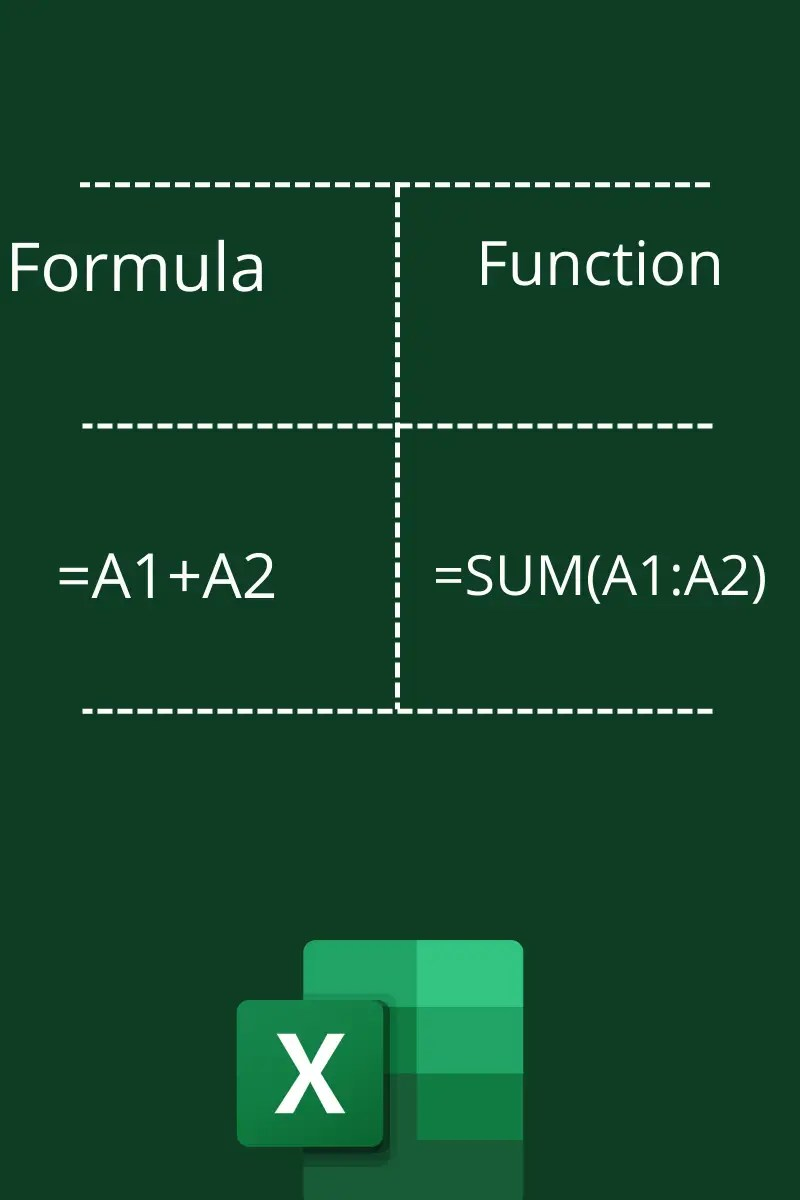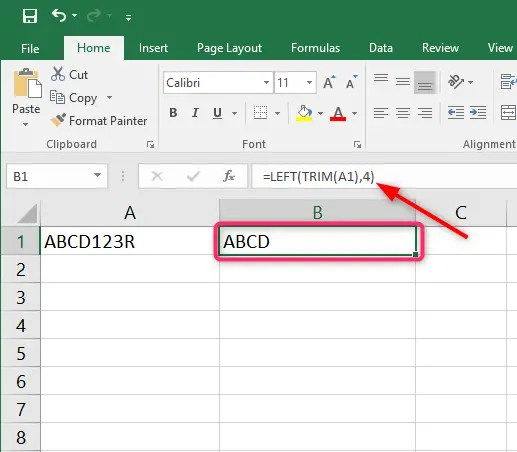# Excel Formula Vs Excel Function

One of the most confusing terms in Microsoft Excel is Function and Formula. Most people confuse these two features because they perform similar tasks.  But there’s a big difference in how they are designed and how they operate. In this article, I will help you understand the differences between Excel  Formulas Vs Excel Function.

## What is an Excel Formula?

An Excel formula is a  mathematical formula that you can formulate to calculate simple calculations. For Instance, you can find the sum of two numbers by typing =5+7; then press enters in a cell. Generally, you can use Excel formulas to add, subtract, multiply or divide.

## What is an Excel Function?

An excel function is a predefined formula that allows users to compute complex maths in Excel. For instance, calculating mortgage rates, loan rates, standard deviation, EMI, maximum purchase, and many more.

## Excel Formula Vs Excel Function

An excel formula refers to a statement that a user writes to be calculated, while an Excel Function refers to a piece of code predefined by Excel to compute specific values, and they are wrapped inside formulas. You can use the Excel functions to create your custom formula. For example, if you enter =MAXIMUM(B1:B90), then you have created a formula using the Maximum function.

## What is the difference between a Function and a Formula in Excel?

1. Users formulate formulas while Functions are predefined in Excel. (Note that you can add new functions using VBA code)
2. Formulas compute basic maths while functions solve complex problems
3. Formulas start with an equal then the problem you need to solve. For example =3+5 . Conversely, An excel function starts with an equals followed by a name and then the problem in parenthesis. For example =Sum(A1:A5)### Examples of Formulas

2. Subtraction: =8-5 or =B-A Subtracts two or more numbers
3. Multiplication =6*9 or  =A*B Multiply two or more numbers
4. Division =10/2 or =A/B Divide two or more number

### Examples of Functions

1. =NOW(). This function returns the current time and date
2. =COUNTIF(). This function counts if a condition is met
3. =MAX() Returns the maxi,u, value in an Excel range
4. =MIN() Returns the minimum value from a range in Excel

## Formula Vs Function comparison table

 Excel Formula Excel Function Defined by user Predefined by Excel It does not feature a parenthesis Features an opening and closing parenthesis Example =A1+A2+A3+A4+A5 Example =SUM(A1:A5)

• Can I create my own function?

You can create your own function using VBA code. Excel allows users to customize their own functions to calculate specific values using Excel. However, you need to give the function a unique name before saving.

• Can I use two functions in one formula?Excel allows users to combine more than two functions to solve complex problems. For example, you can combine the LEFT function and the TRIM function to delete the last four values of a string.

## Conclusion

It’s easy to tell the difference between Excel formula Vs Excel function. All you need to look is the parenthesis. A formula has no parenthesis while a function has a an opening and closing parenthisias. The only time a formula features a parenthisis is when you you use a function within a formula.